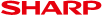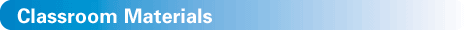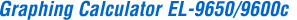Handbook vol.1 (Algebra)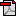Handbook vol.1 (Algebra) for EL-9650/9600c/9450/9400 (1.4M)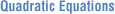Slope and Intercept of Quadratic Equations (72K)Shifting a Graph of Quadratic Equations (48K)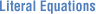Solving a Literal Equation Using the Equation Method (Amortization) (48K)Solving a Literal Equation Using the Graphic Method (Volume of a Cylinder) (48K)Solving a Literal Equation Using Newton's Method (Area of a Trapezoid) (48K)Graphing Polynomials and Tracing to Find the Roots (56K)Graphing Polynomials and Jumping to Find the Roots (56K)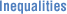Solving Inequalities (56K)Solving Double Inequalities (44K)System of Two-Variable Inequalities (40K)Graphing Solution Region of Inequalities (56K)Slope and Intercept of Absolute Value Functions (48K)Shifting a Graph of Absolute Value Functions (60K)Solving Absolute Value Equations (32K)Solving Absolute Value Inequalities (60K)Evaluating Absolute Value Functions (44K)Graphing Rational Functions (52K)Solving Rational Function Inequalities (40K)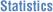Statistics calculations (48K)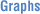Rectangular coordinate graphs (38K)Polar coordinate graphs (40K)Substitute graph function (40K)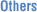CALC function (41K)Original slide show (52K)

 Handbook vol.2 (Programmes)Handbook vol.2 (Programmes) for EL-9650/9600c/9450/9400 (692K)

 Resource BookPre-Algebra/Algebra for EL-9600 (376K)*Pre-Calculus/Calculus for EL-9600 (392K)*Trigonometry for EL-9600 (368K)*Statistics for EL-9600 (368K)*

* The EL-9650/9600c has the same functions as the EL-9600.